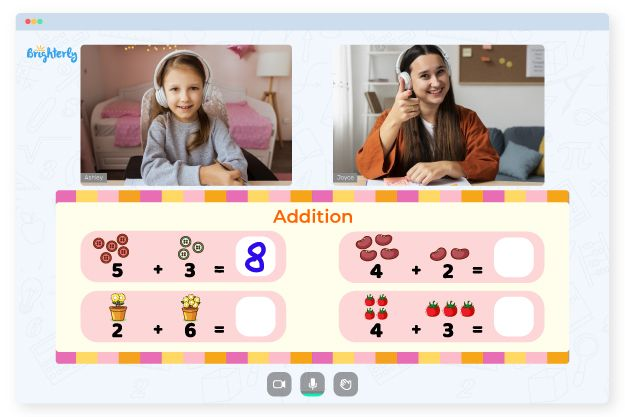# Repeated Addition – Definition, Examples, Practice Problems, FAQs

Let’s embark on a fun mathematical journey where we’ll explore a foundational concept known as Repeated Addition. The term may sound like a complex arithmetic term but in reality, it’s pretty straightforward and a lot of fun. Here, we are going to break down this concept in the easiest possible way so that our young mathematicians at Brighterly can understand and enjoy it.

Repeated Addition refers to the process of adding the same number over and over again. It’s one of the first steps in understanding multiplication, making it a fundamental cornerstone in math.

Here’s a simple illustration. Imagine you have 3 bags and each bag contains 4 apples. If you add the apples in all the bags, you’ll perform a repeated addition. Like so: 4 apples + 4 apples + 4 apples.

## Connection of Repeated Addition with Multiplication

Repeated addition is a stepping stone to understanding multiplication. Multiplication is essentially a quick way of performing repeated addition. For instance, 4 times 3 (4 x 3) is the same as adding 3, four times (3+3+3+3). This understanding helps to simplify complex calculations and accelerates our math skills.

## How to Teach Repeated Addition

Teaching repeated addition can be made fun and engaging with these simple methods:

1. Use of Objects: Use physical items like blocks, marbles, or buttons. Start by grouping them and then add each group. This method works well as it provides a visual and hands-on approach.

2. Drawings: Encourage children to draw pictures representing the groups of objects being added repeatedly. This promotes understanding and retention.

3. Word Problems: Create real-life scenarios that require repeated addition to solve. This not only builds math skills but also helps children see how math applies in everyday life.

Let’s dive into some practical examples to grasp the concept of repeated addition:

1. Example 1: You have 5 candies and you want to distribute them equally to 4 friends. How many candies does each friend get? In this case, you’d add 5 candies 4 times (5+5+5+5) to get the total number of candies you distributed.

2. Example 2: Suppose you are helping in a bakery and need to pack 3 boxes of 6 cookies each. The total number of cookies would be calculated using repeated addition as (6+6+6).

## Practice Problems

Ready to give it a try? Let’s practice some problems together!

1. You have 4 sets of lego blocks, each containing 7 blocks. How many blocks do you have in total?
2. You want to water 5 plants and each plant needs 3 cups of water. How many cups of water do you need in total?

1. 4 sets of lego blocks times 7 equals 28 (7+7+7+7).
2. 5 plants times 3 cups of water equals 15 (3+3+3+3+3).

## Conclusion

Understanding repeated addition is a crucial step in a child’s mathematical development. It lays the groundwork for learning multiplication and other crucial arithmetic operations. With consistent practice, perseverance, and the right resources, any child can master the concept of repeated addition, and even find joy in the process!

At Brighterly, we are committed to making math fun and accessible for every child. Our comprehensive and child-friendly learning resources simplify complex concepts like repeated addition and turn them into engaging learning experiences. We understand that each child has a unique learning style and pace, and our adaptive learning tools cater to these individual needs.

Our virtual platform creates a safe and stimulating environment for children to explore various math concepts. The interactive lessons, coupled with practical exercises, enable children to grasp and apply their knowledge effectively. We believe in turning challenges into opportunities for learning and growth.

### Is repeated addition the same as multiplication?

Yes, repeated addition is the foundation of multiplication. It’s a simpler way to understand multiplication. For instance, 4 times 3 is the same as adding 3 four times (3+3+3+3).

### How can repeated addition help in everyday life?

Understanding repeated addition can help solve everyday problems, such as calculating the total cost of multiple identical items, determining the amount of food needed for multiple pets, or even figuring out how much time it takes to complete identical tasks multiple times.

Information Source:• An online tutor could provide the necessary support.

Does your child need extra help with mastering addition basics? Start studying with an online tutor.